IMO Shortlist 2009 problem G8

Kvaliteta:
Avg: 3,0
Težina:
Avg: 9,0
Let$ABCD$ be a circumscribed quadrilateral. Let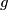$g$ be a line through$A$ which meets the segment$BC$ in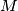$M$ and the line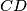$CD$ in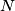$N$. Denote by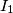$I_1$,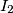$I_2$ and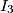$I_3$ the incenters of$\triangle ABM$,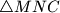$\triangle MNC$ and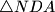$\triangle NDA$, respectively. Prove that the orthocenter of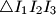$\triangle I_1I_2I_3$ lies on$g$.

Proposed by Nikolay Beluhov, Bulgaria
Izvor: Međunarodna matematička olimpijada, shortlist 2009